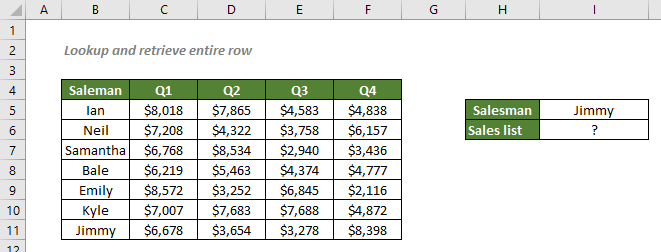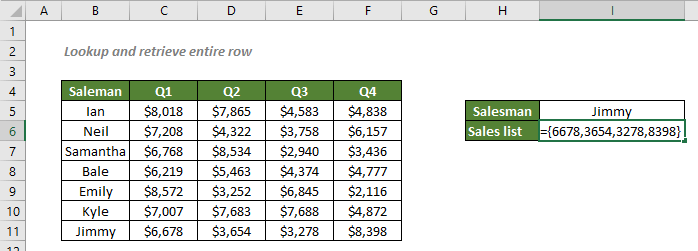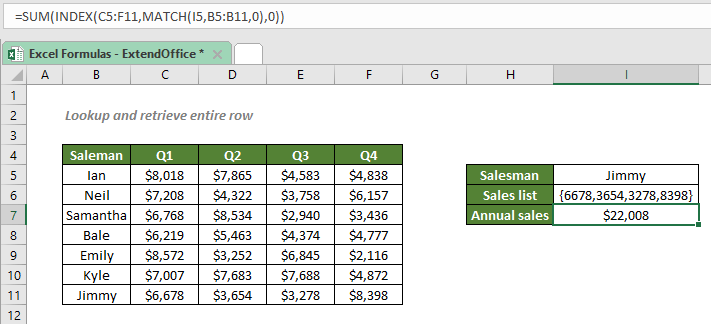## Lookup and retrieve entire row

To lookup and retrieve an entire row of data by matching a specific value, you can use the INDEX and MATCH functions to create an array formula.#### Lookup and retrieve an entire row based on a specific value

To get the list of sales made by Jimmy according to the table above, you can first use the MATCH function to return the position of the sales made by Jimmy, and which will be fed to INDEX to retrieve the values at the position.

#### Generic syntax

=INDEX(return_range,MATCH(lookup_value,lookup_array,0),0)

√ Note: This is an array formula that requires you to enter with Ctrl + Shift + Enter.

• return_range: The range that contains the entire row you want to return. Here refers to the sales range.
• lookup_value: The value the combination formula used to find its corresponding sales information. Here refers to the given salesman.
• lookup_array: The range of cells where to match the lookup_value. Here refers to the name range.
• match_type 0: Forces MATCH to find the first value that exactly equals to the lookup_value.

To get the list of sales made by Jimmy, please copy or enter the formula below in the cell I6, press Ctrl + Shift + Enter, and then double click on the cell and press F9 to get the result:

=INDEX(C5:F11,MATCH("Jimmy",B5:B11,0),0)

Or, use a cell reference to make the formula dynamic:

=INDEX(C5:F11,MATCH(I5,B5:B11,0),0)#### Explanation of the formula

=INDEX(C5:F11,MATCH(I5,B5:B11,0),0)

• MATCH(I5,B5:B11,0): The match_type 0 forces the MATCH function to return the position of Jimmy, the value in I5, in the range B5:B11, which is 7 since Jimmy is at the 7th position.
• INDEX(C5:F11,MATCH(I5,B5:B11,0),0) = INDEX(C5:F11,7,0): The INDEX function returns all the values in the 7th row of the range C5:F11 in an array like this: {6678,3654,3278,8398}. Note that to make the array visible in Excel, you should double click on the cell where you entered the formula, and then press F9.

#### Sum an entire row up based on a specific value

Now that we have all the sales information made by Jimmy, to get the annual sales volume made by Jimmy, we can just add the SUM function to the formula to sum all the sales values from the list.

#### Generic syntax

=SUM(INDEX(return_range,MATCH(lookup_value,lookup_array,0),0))

In this example, to get the annual sales volume made by Jimmy, please copy or enter the formula below in the cell I7, and press Enter to get the result:

=SUM(INDEX(C5:F11,MATCH(I5,B5:B11,0),0))#### Explanation of the formula

=SUM(INDEX(C5:F11,MATCH(I5,B5:B11,0),0))

• MATCH(I5,B5:B11,0): The match_type 0 forces the MATCH function to return the position of Jimmy, the value in I5, in the range B5:B11, which is 7 since Jimmy is at the 7th position.
• INDEX(C5:F11,MATCH(I5,B5:B11,0),0) = INDEX(C5:F11,7,0): The INDEX function returns all the values in the 7th row of the range C5:F11 in an array like this: {6678,3654,3278,8398}. Note that to make the array visible in Excel, you should double click on the cell where you entered the formula, and then press F9.
• SUM(INDEX(C5:F11,MATCH(I5,B5:B11,0)),0) = SUM({6678,3654,3278,8398}): The SUM function sums all the values in the array, and then gets the annual sales volume made by Jimmy, \$22,008.

#### Further analysis of an entire row based on a specific value

For additional processing on the sales made by Jimmy, you can simply add other functions such as SUM, AVERAGE, MAX, MIN, LARGE, etc. to the formula.

For example, to get the average of Jimmy’s sales for each quarter, you can use the formula:

=AVERAGE(INDEX(C5:F11,MATCH(I5,B5:B11,0),0))

To find out the lowest sales made by Jimmy, use one of the below formulas:

=MIN(INDEX(C5:F11,MATCH(I5,B5:B11,0),0))
OR
=SMALL(INDEX(C5:F11,MATCH(I5,B5:B11,0),0),1)

#### Related functions

Excel INDEX function

The Excel INDEX function returns the displayed value based on a given position from a range or an array.

Excel MATCH function

The Excel MATCH function searches for a specific value in a range of cells, and returns the relative position of the value.

#### Related Formulas

Lookup and retrieve entire column

To lookup and retrieve an entire column by matching a specific value, an INDEX and MATCH formula will do you a favor.

Exact match with INDEX and MATCH

If you need to find out the information listed in Excel about a specific product, movie or a person, etc., you should make a good use of the combination of INDEX and MATCH functions.

Approximate match with INDEX and MATCH

There are times when we need to find approximate matches in Excel to evaluate employees' performance, grade students’ scores, calculate postage based on weight, etc. In this tutorial, we will talk about how to use the INDEX and MATCH functions to retrieve the results we need.

Case-sensitive lookup

You may know that you can combine the INDEX and MATCH functions, or use the VLOOKUP function to lookup values in Excel. However, the lookups aren’t case-sensitive. So, in order to perform a case-sensitive match, you should take advantage of the EXACT and CHOOSE functions.

### The Best Office Productivity Tools

#### Kutools for Excel - Helps You To Stand Out From Crowd

 Popular Features: Find, Highlight or Identify Duplicates  |  Delete Blank Rows  |  Combine Columns or Cells without Losing Data  |  Round without Formula ... Super VLookup: Multiple Criteria  |  Multiple Value  |  Across Multi-Sheets  |  Fuzzy Lookup... Adv. Drop-down List: Easy Drop Down List  |  Dependent Drop Down List  |  Multi-select Drop Down List... Column Manager: Add a Specific Number of Columns  |  Move Columns  |  Toggle Visibility Status of Hidden Columns  |  Compare Columns to Select Same & Different Cells ... Featured Features: Grid Focus  |  Design View  |  Big Formula Bar  |  Workbook & Sheet Manager | Resource Library (Auto Text)  |  Date Picker  |  Combine Worksheets  |  Encrypt/Decrypt Cells  |  Send Emails by List  |  Super Filter  |  Special Filter (filter bold/italic/strikethrough...) ... Top 15 Toolsets:  12 Text Tools (Add Text, Remove Characters ...)  |  50+ Chart Types (Gantt Chart ...)  |  40+ Practical Formulas (Calculate age based on birthday ...)  |  19 Insertion Tools (Insert QR Code, Insert Picture from Path ...)  |  12 Conversion Tools (Numbers to Words, Currency Conversion ...)  |  7 Merge & Split Tools (Advanced Combine Rows, Split Excel Cells ...)  |  ... and more

Kutools for Excel Boasts Over 300 Features, Ensuring That What You Need is Just A Click Away...#### Office Tab - Enable Tabbed Reading and Editing in Microsoft Office (include Excel)

• One second to switch between dozens of open documents!
• Reduce hundreds of mouse clicks for you every day, say goodbye to mouse hand.
• Increases your productivity by 50% when viewing and editing multiple documents.
• Brings Efficient Tabs to Office (include Excel), Just Like Chrome, Edge and Firefox.